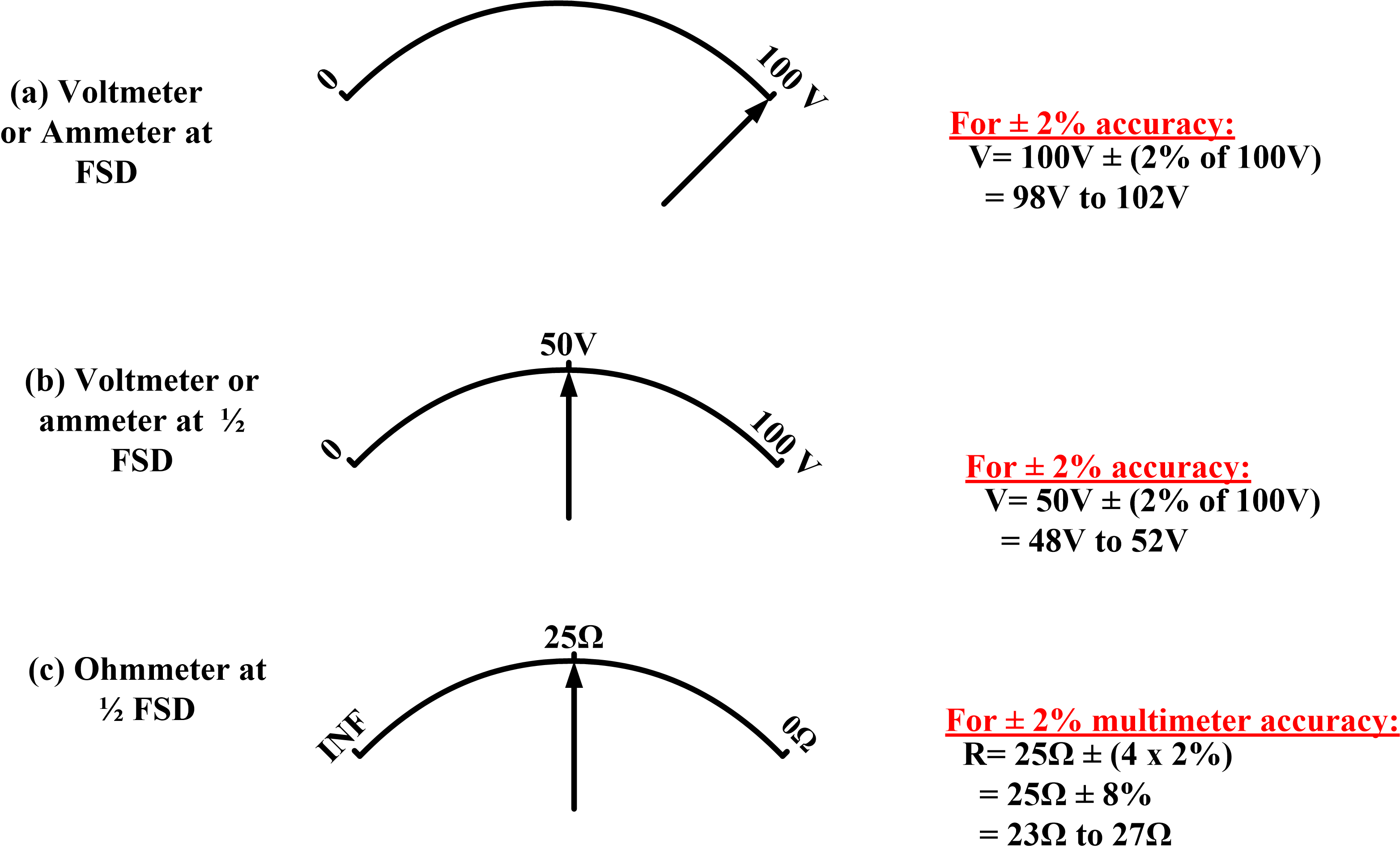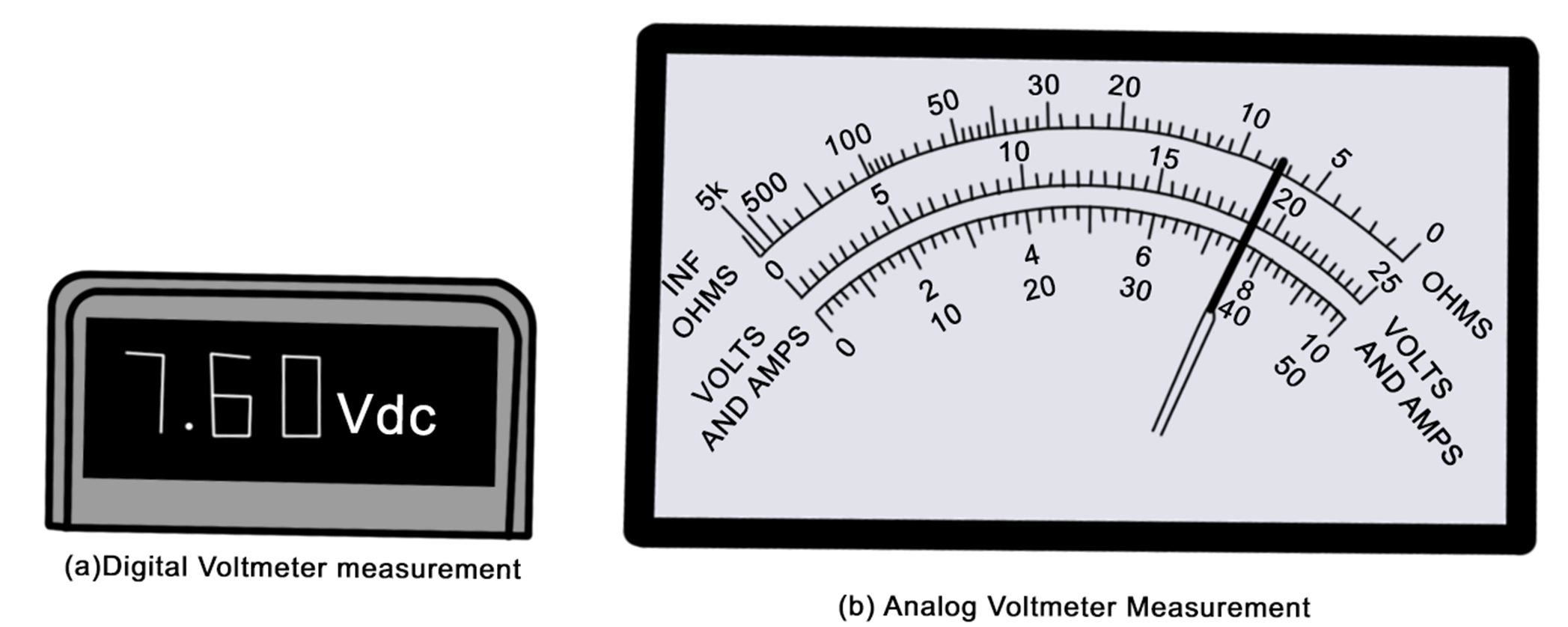Home / Instrumentation / Accuracy and Precision in Measurement | Difference between Accuracy and Precision

# Accuracy and Precision in Measurement | Difference between Accuracy and Precision

Want create site? Find Free WordPress Themes and plugins.

## Analog Meter Accuracy

The accuracy of all deflection instruments is specified by the manufacturer as a percentage of full-scale deflection (FSD).

For example, a voltmeter with an FSD of 100 V might have its accuracy stated as ±2%. In this case, the maximum possible error at all points on the scale is ±2% of 100 V or ±2 V. Thus when the pointer is indicating exactly at 100 V, as shown in figure 1 (a), the measured voltage is correctly stated as 100 V ±2 V, or 98 V to 102 V. the actual level of the measured voltage might be anywhere from 98 V to 102 V.

When the pointer of this voltmeter indicates 50 V, as illustrated in figure 1 (b), the actual measured voltage must be taken as 50 V ± (2% of full-scale deflection), or 50 V ± 2 V. This means that the measured voltage is somewhere between 48 V and 52 V. The ±2 V error is ±4% of 50 V. Consequently, the measurement percentage error increases as the pointer moves further away from FSD.Fig.1: Accuracy in Deflection Instruments

Deflection-type multimeters typically have an accuracy of ±2 % for voltage and current measurements, although more accurate instruments are available. As illustrated in figure 1 (c), the error becomes considerably greater when the instrument is used for resistance measurements. Ohmmeter measurements are most accurate when the pointer is near mid-scale. However, even at mid-scale, the resistance measurement error is typically ± (4x % of mid-scale resistance), where x is the multimeter specified accuracy. Thus, for a multimeter with an accuracy of ±2 %, the mid-scale accuracy of the ohmmeter function is ± (8 % of mid-scale resistance). When the measurements are made away from mid-scale, the error is increased.

## Digital Instrument Accuracy

The accuracy of digital multimeter might be stated by the manufacturer as ±0.5 % Reading ± 1 digit.

This means ± (0.5 % of the reading) ± 1 digit, where the 1 digit refers to the right-hand numeral of the display. For example, a display of 100.0 V would be accurate to:

±(0.5 % of 100.0 V)±000.1V

$=\pm (0.5V)\pm 000.1V$

$=\pm 0.6V$

Therefore, the measured voltage could be stated as:

$100.0V\pm 0.6V=99.4V\text{ }to\text{ }100.6V$

## Precision

The precision with which a measurement is made by an instrument is not the same as the accuracy of measurement, although accuracy and precision are related.

The precision can be defined as the smallest change that can be detected in a measured quantity.

Consider the digital voltmeter indication shown in figure 2 (a).  For the 7.60 V indicated quantity, a 1 on the last (right side) numeral represents 10 mV. If the measured quantity increases or decreases by 10 mV, the reading becomes 7.61 V or 7.59 V, respectively. A voltage change smaller than 10 mV is unlikely to produce a change in the measured quantity. Therefore, 10 mV is the smallest voltage change that can be detected in this case. So, it can be stated that the voltage is measured with a precision of 10 mV.Fig.2: Precision of Measurement in an Analog and Digital Instruments

For analog voltmeter in figure 2 (b), the pointer position on the 10 V range can be read within one-half of the smallest scale division. Since the smallest scale division represents 0.2 V (on the 10 V range), one-half of the scale division is 0.1 V. So, 0.1 V is the smallest detectable change in this case, or the measurement precision of this analog instrument on the 10 V range is 0.1 V.

Suppose the digital voltmeter in figure 2 has an accuracy of ± (0.5 %±1 d). The measured voltage is 7.60 V ± (0.5 %±10 mV), or 7.60 V ± 48 mV; meaning that the actual voltage is somewhere between 7.648 V and 7.552 V. So, although the quantity is measured with a precision of 10 mV, the measurement accuracy is ±48 mV.

The analog voltmeter in figure 2 (b) might have a typical accuracy of ±2% of full scale, or ±2% of 10 V, which is ±0.2V. Thus, the measured quantity is 7.60 V ± 0.2 V; that is, 7.4 V to 7.8 V. in this case, the measurement is made to a precision of 0.1 V, but the measurement accuracy is ±0.2V.

## Basic Electrical Instruments Characteristics

 Instrument Quantity measured Instrument resistance Instrument connection Voltmeter Voltage Very high In parallel with the points at which the voltage is to be measured Ammeter Electric Current Very low In series with the circuit in which current is to be measured Ohmmeter Resistance Depends on range settings In parallel with the component that is to have its resistance measured
Did you find apk for android? You can find new Free Android Games and apps.

### About Ahmad FaizanMr. Ahmed Faizan Sheikh, M.Sc. (USA), Research Fellow (USA), a member of IEEE & CIGRE, is a Fulbright Alumnus and earned his Master’s Degree in Electrical and Power Engineering from Kansas State University, USA.